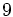# Cyclic group:Z9

View a complete list of particular groups (this is a very huge list!)[SHOW MORE]

## Definition

This group, denoted$C_9, \Z_9, \Z/9\Z$, is defined as the cyclic group of order$9$.

## Arithmetic functions

Function Value Explanation
order 9
exponent 9
derived length 1
Frattini length 2
Fitting length 1
subgroup rank 1

## Group properties

Property Satisfied Explanation
cyclic group Yes
elementary abelian group No
abelian group Yes
group of prime power order Yes
nilpotent group Yes
solvable group Yes

## GAP implementation

### Group ID

This finite group has order 9 and has ID 1 among the groups of order 9 in GAP's SmallGroup library. For context, there are groups of order 9. It can thus be defined using GAP's SmallGroup function as:

SmallGroup(9,1)

For instance, we can use the following assignment in GAP to create the group and name it$G$:

gap> G := SmallGroup(9,1);

Conversely, to check whether a given group$G$ is in fact the group we want, we can use GAP's IdGroup function:

IdGroup(G) = [9,1]

or just do:

IdGroup(G)

to have GAP output the group ID, that we can then compare to what we want.

### Other descriptions

The group can be described using GAP's CyclicGroup function:

CyclicGroup(9)